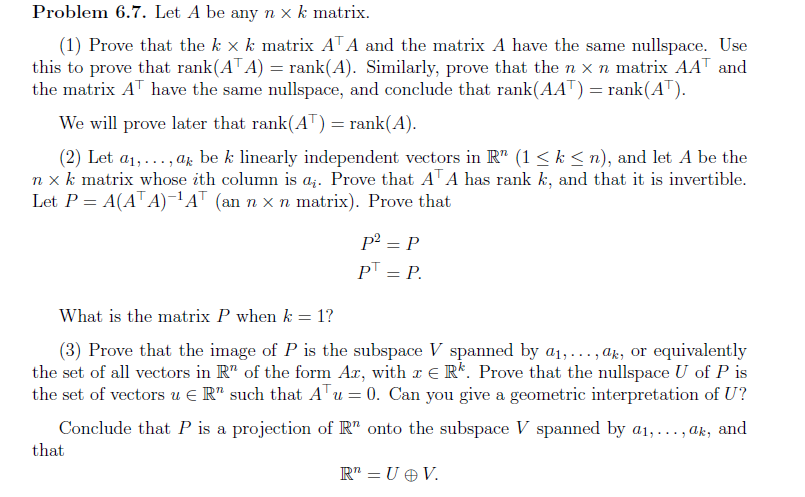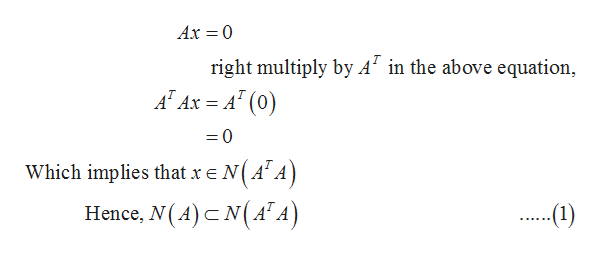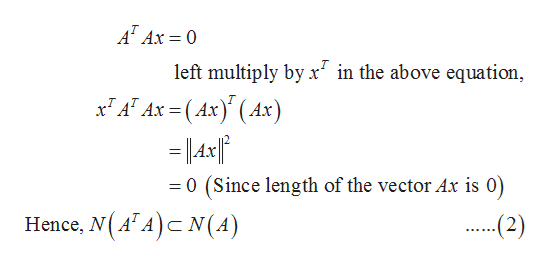# Problem 6.7. Let A be any nx k matrix(1) Prove that the k x k matrix ATA and the matrix A have the same nullspace. Usethis to prove that rank(ATA) = rank(A). Similarly, prove that the n x n matrix AAT andthe matrix AT have the samenullspace, and conclude that rank(AAT) =rank(AT)We will prove later that rank(AT) = rank(A)(2) Let a1,.. ., a be k linearly independent vectors in R" (1 k

Question
12 views

Please, help me with a step by step solutions to this problem and I will be very grateful to you. I don't have a strong background in algebra. I hope you will help.help_outlineImage TranscriptioncloseProblem 6.7. Let A be any nx k matrix (1) Prove that the k x k matrix ATA and the matrix A have the same nullspace. Use this to prove that rank(ATA) = rank(A). Similarly, prove that the n x n matrix AAT and the matrix AT have the same nullspace, and conclude that rank(AAT) =rank(AT) We will prove later that rank(AT) = rank(A) (2) Let a1,.. ., a be k linearly independent vectors in R" (1 k
check_circle

Step 1

Since we only answer up to first question, we’ll answer the first 1. Please resubmit the question and specify the other squestions (up to 1) you’d like answered.

1.A is the matrix of order n*k and tr(A). A is the matrix of order k*k.

Null space of matrix A is defined as {x : x  belongs to R(n-tuple) and Ax=0}

Such that N(A)= {x : x  belongs to R(n-tuple) and Ax=0}

Consider any x which belongs to null apace of A. Thenhelp_outlineImage TranscriptioncloseAx 0 right multiply by A in the above equation AT Ax A (0) =0 Which implies that x e NAA Hence, N(A) N(ATA ...1) fullscreen
Step 2

Now, consider any x which belongs to null space of tr(A). A, thenhelp_outlineImage TranscriptioncloseA Ax 0 left multiply by x in the above equation Ax(Ax(Ax) 0 (Since length of the vector Ax is 0) Hence, N(AA) N(A) (2) fullscreen
Step 3

By equations (1) and (2) it is clear that null space A and n...

### Want to see the full answer?

See Solution

#### Want to see this answer and more?

Solutions are written by subject experts who are available 24/7. Questions are typically answered within 1 hour.*

See Solution
*Response times may vary by subject and question.
Tagged in

### Math